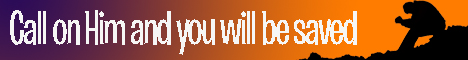Note:  Do not rely on this information. It is very old.

# Hydrostatics

Hydrostatics, the science of the force-relations of liquids in equilibrium. A liquid has no rigidity - that is to say, it may change its shape under the action of extremely small forces. This absence of rigidity may be difficult to observe in some cases, owing to the viscosity of the liquids taken. Nevertheless, with all liquids small forces can produce indefinite distortion, although in the case of viscous liquids much time may be required for the effect to be rendered visible. Water, ether, and alcohol are examples of mobile liquids; pitch and sealing-wax are examples of very viscous liquids. A stick of sealing-wax supported horizontally at its extremities will in the course of weeks become so curved that it falls from its supports. A tallow candle if similarly supported will at once manifest a certain amount of curvature, but will not become further bent. The sealing wax is called a hard liquid; the candle is a soft solid. The resistance of a liquid to diminution of bulk is very great, like that of a solid. The compressibility of water, for example, is so slight that for a long time water was thought incompressible. [Cavendish Experiment.] This characteristic distinguishes liquids from gases, which possess great elasticity (i.e. they change their volume readily under the action of very small forces) as well as no rigidity. If a mass of liquid be at rest its pressure at any point on any surface must be at right angles to that surface, otherwise the oblique pressure of a non-rigid substance would produce motion. Hence it may be shown that at any point the pressure is the same in all directions, and an Ordinary definition of a liquid at once follows, which assigns to such a substance that characteristic property. If therefore a pressure of a definite intensity per square inch be imposed at one spot on an enclosed volume of liquid, that pressure will be transmitted in all directions through the liquid, and every portion of its boundary will feel the same intensity of pressure. This is the principle of the hydraulic press, and the explanation of the hydrostatic paradox (q.v.).

When a solid body is entirely immersed in water (this liquid is taken as, an example) every portion of its surface is acted upon by a normal pressure.

An infinite number of forces thus exist, and we know that their resultant is an upward force equal to the weight of the water displaced, for before the body was introduced the water subsequently displaced was just supported by the same set of forces. Hence the foreign substance is acted upon downweirds by its own weight and upwards by the weight of water displaced. If it has the same density (q.v.) as water these two forces balance, and the body remains in position without the application of other forces. If its own weight preponderates its density is said to be greater than unity, and it shows a tendency to sink. If the water displaced has the greater weight its density is less than unity, and it tends to rise to the surface.

If this tendency is permitted it will assume a floating position such that the weight of the water displaced in that position is now equal to the weight of the substance itself.

Further, it then assumes a position such that its centre of gravity and that of the water displaced are in the same vertical line, otherwise from statical considerations there could be no equilibrium. The density of a substance, or relative mass of the substance compared with an equal volume of water, is calculable when we know its weight in air and in water. The difference between its weight in air and in a vacuum is generally neglected. It is then only necessary to divide the weight in air by the loss of weight in water. If it float in water, the weight of water displaced by the whole volume is determined by attaching a heavy substance to it, such that the combination will sink. The whole is then estimated as one body and a deduction made for the heavy substance.

The free surface level of a liquid at rest must be horizontal. For a spherical particle at the surface is acted upon by its own weight and by a hemisphere of fluid. The former is a vertical force, and the resultant of the latter must therefore also be vertical. This could not be unless the surface were at right angles to the vertical.

The pressure at any depth in a liquid is that due to the vertical height of liquid above. It may be that the base of the vessel is greater or less in area than the surface of the liquid; the total pressure on the base will be independent of such variety if the depth of liquid remains constant. Thus clear distinction must be made between the weight of the liquid and the total pressure of liquid on the base of the vessel.